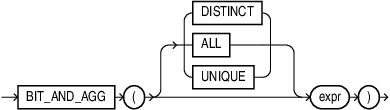## BIT_AND_AGG

SyntaxDescription of the illustration bit_and_agg.eps

Purpose

` BIT_AND_AGG` is a bitwise aggregation function that returns the result of a bitwise AND operation.

You can use ` BIT_AND_AGG` as part of a `GROUP` `BY` query, window function, or as an analytical function. The return type of `BIT_AND_AGG` is always a number.

Semantics

The keywords `DISTINCT` or `UNIQUE` ensure that only unique values in `expr` are used for computation. `UNIQUE` is an Oracle-specific keyword and not an ANSI standard.

NULL values in the `expr` column are ignored.

Returns NULL if all rows in the group have NULL `expr` values.

Floating point values are truncated to the integer prior to aggregation. For instance, the value 4.64 is converted to 4, and the value 4.4 is also converted to 4.

Negative numbers are represented in two’s complement form internally prior to performing an aggregate operation. The resultant aggregate could be a negative value.

Range of inputs supported: -2 raised to 127 to (2 raised to 127) -1

Numbers are internally converted to a 128b decimal representation prior to aggregation. The resultant aggregate is converted back into an Oracle Number.

For a given set of values, the result of a bitwise aggregate is always deterministic and independent of ordering.

Example 7-2 Use the BIT_AND_AGG Function

Select two numbers and their bitwise representation:

``````SELECT '011' num, bin_to_num(0,1,1) bits FROM dual
UNION ALL SELECT '101' num, bin_to_num(1,0,1) bits FROM dual;

NUM       BITS
--- ----------
011          3
101          5``````

Perform the bitwise AND operation:

``````SELECT bit_and_agg(bits)
FROM (SELECT '011' num, bin_to_num(0,1,1) bits FROM dual
UNION ALL SELECT '101' num, bin_to_num(1,0,1) bits FROM dual);

BIT_AND_AGG(BITS)
-----------------
1``````

Only the first bit is identical in both rows, thus the result is 001, which is the number 1.